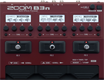# Factory : Flamenco

Discussion in 'Zoom B3n' started by Tonelib, May 20, 2018.

1. Factory : FlamencoDevice: Zoom B3n
Firmware: 2.00

Name on device: Flamenco
Optimized for: Phones/Speaker

Effects chain:This sound matches rasgueado style playing for flamenco.

Effect: "MB Comp" (Dynamics), active - "yes"
"Comp" = 80
"LoTHR" = 64
"HiTHR" = 58
"Volume" = 40

Effect: "Exciter" (Filter), active - "yes"
"Bass" = 0
"Treble" = 59
"Volume" = 70
"ON/OFF" = LATCH

Effect: "BassGEQ" (Filter), active - "yes"
"50Hz" = -10.0
"120Hz" = -5.0
"400Hz" = -2.5
"500Hz" = 0.0
"800Hz" = 2.0
"4.5kHz" = 5.0
"10kHz" = 5.5
"Volume" = 80
"50Hz" = -9.0
"120Hz" = 0.0
"400Hz" = 0.0
"500Hz" = 0.5
"800Hz" = 5.5
"4.5kHz" = 3.5
"10kHz" = 2.5
"Volume" = 80
"Parameter set" = A

Effect: "Ba Detune" (Modulation), active - "yes"
"Cent" = 20
"PreD" = 10
"Tone" = 10
"Mix" = 66

Effect: "Room" (Reverb), active - "yes"
"PreD" = 38
"Decay" = 12
"Mix" = 44
"Tail" = Off

Patch Volume: 85